Reach Us+44-1522-440391
Inflation Forecasting in Ghana-Artificial Neural Network Model Approach | OMICS International
International Journal of Economics & Management Sciences
All submissions of the EM system will be redirected to Online Manuscript Submission System. Authors are requested to submit articles directly to Online Manuscript Submission System of respective journal.

# Inflation Forecasting in Ghana-Artificial Neural Network Model Approach

Yusif M Hadrat1, Eshun Nunoo Isaac K2 and Effah Sarkodie Eric3*

1Department of Economics, Kwame Nkrumah University of Science and Technology, Kumasi, Ghana

2Ghana College, Kumasi, Ghana

3University of Education, Winneba, College of Technology Education, Department of Accounting Studies Education, Kumasi, Ghana

*Corresponding Author:
Effah Sarkodie Eric
M. Phil. Economics, B. A. Economics
University of Education, Winneba
College of Technology Education
Department of Accounting Studies Education,Kumasi, Ghana
Tel: 03220-53602
E-mail: [email protected]

Received Date: July 14, 2015; Accepted Date: July 30, 2015; Published Date: August 05, 2015

Citation: Hadrat YM, Eshun Nunoo Isaac K, Eric ES (2015) Inflation Forecasting in Ghana-Artificial Neural Network Model Approach. Int J Econ Manag Sci 4:274. doi:10.4172/2162-6359.1000274

Copyright: © 2015 Hadrat YM, et al. This is an open-access article distributed under the terms of the Creative Commons Attribution License, which permits unrestricted use, distribution, and reproduction in any medium, provided the original author and source are credited.

Visit for more related articles at International Journal of Economics & Management Sciences

#### Abstract

Artificial Neural Network (ANN) is a modelling technique which is based on the way the human brain process information. ANNs have proved to be good forecasting models in several fields including economics and finance. The ANN methodology is used by some central banks to predict various macroeconomic indicators such as the inflation, money supply, GDP growth etc. The use of the ANN for prediction is common in the forecasting literature but rare in Ghana. This paper forecasts inflation with the ANN method using the Ghanaian data. The monthly y-o-y data between 1991:01 and 2010:12 are used to estimate and forecast for the period 2011:01 to 2011:12. The result of the ANNs are also compared with traditional time series models such as the AR (12) and VAR (14) which use the same set of variables. The basis of comparison is the out-of-sample forecast error (RMSFE). The results show that the RMSFE of the ANNs are lower than their econometric counterparts. That is, by this comparative criterion forecast based on ANN models are more accurate.

#### Keywords

Inflation; Artificial neural network; Demand; Supply

#### Introduction

Price stability is one of the key objectives of monetary policy. To achieve such mandate, policy makers need to be forward-looking. This calls for good inflation forecasting ability for the monetary authorities. The inflation forecast is used to guide policy discussions in determining the appropriate policy stance to be adopted. In the case of an inflation targeting regime, the inflation forecast can alert policymakers to take policy response should the forecast indicate that the inflation outlook may deviate for the target. Hence, accurately forecasting the inflation is crucial for the monetary authorities.

Artificial Neural Network (ANN) model is currently a popular forecasting technique in several fields such computer science, engineering, economics, finance etc. ANNs have been used to predict variables such as bond prices, exchange rates, stock returns, money supply, electricity demand, construction demand, inflation rates etc. [1,2]. In the prediction of the inflation, in particular, scores of studies have compared the inflation forecasting performance of traditional econometric models with the neural network models and have concluded that the ANNs outperform the econometric models in the prediction of inflation [3-7].

Some central banks including inflation-targeting central banks like The State Bank of Pakistan, CZECH National Bank, Bank of Canada and Bank of Jamaica use forecasting models which are based on ANN methodology for predicting various macroeconomic indicators, like inflation, GDP growth and money supply etc [8-12]. The main purpose of this paper is to use the ANN technique to forecast inflation in Ghana for the period 2011: 01–2011:12 using the data between 1991: 01 and 2010:12.The forecast performance of the ANNs is also compared with their counterpart traditional models, AR (12) and VAR (14). The results show that the ANNs predict accurately than the econometric models.

The paper hereafter is organised in four sections. Section two presents the neural network methodology. Section three also provides the data type and source and the model specifications. The empirical results are presented in section four and the last section presents the summary of the findings.

#### Methodology

The Artificial Neural Network methodology was developed in an effort to model the way the human brain process information. The human brain learns by experience: It receives information and recognises the pattern; the brain then generalises and is able to predict based on the information received. It is this way of information processing by the brain that the ANN model tends to mimic. Although ANN models are too far from the way the human brain performs, by mimicking the basic features of the biological neural networks, they have succeeded in doing certain jobs very well [13-15].

The human neuron

The human brain or the central nervous system is made up of interconnected units called neurons. This system or group of interconnected neurons working together to perform the functions of the brain (i.e., learning) is the neural network. By definition, “Neurons are basic signalling units of the nervous system of a living being in which each neuron is a discrete cell whose several processes are from its cell body.” Figure 1 shows the biological structure of the human neuron.

The biological neuron has four main regions to its structure: The cell body, the dendrites or membrane, the axon and the synapses. The cell body is the heart of the neuron. The human neuron receives signals through synapses located on the dendrites or membrane. When the signals received is strong enough (i.e., surpasses a certain threshold), the cell body is activated and emits another signal through the axon. The emitted signal (or action potentials) is sent to activate other neurons within the system. As similar signals continue to cross the threshold, the network recognises the path of the signals, assumes a pattern, and as a result generalises that if the signal is like this then, the output should be that. That is, the network is able to predict based on the pattern of the signals received.

The artificial neuron

The artificial neuron is a mimic of the natural human neuron. The human brain, for example, contains approximately ten billion (1010) neurons, each connected on average to ten thousand (104) other neurons, making a total of 1015 synaptic connections [16-18]. A mimic of the way biological networks perform may appear more than complex. Artificial neural networks represent an attempt at a very basic level to imitate the type of nonlinear learning that occurs in the networks of neurons found in nature.

As shown in Figure 2, a natural neuron uses the synapses located on the dendrite to gather inputs (signals) from other neurons and combines the input information, generate a nonlinear response (“firing”) when some threshold is reached, which it sends to other neurons using the axon. Similarly, the artificial neuron collects inputs (xi) from input neurons, attaches weights and combines them through a combination function such as summation (Σ). It is then activated by a function (usually nonlinear) to produce an output response (y), which is again sent to other neurons [19-24].

The mathematical model

There are three distinct functional operations that take place in a neuron. These are: the weight function, the net input function and the transfer function as shown in Figure 3.

The weight function: First, the inputs, (x: x1, x2,...,xr) are fed into the neuron. Each input is multiplied by a random weight (wi) to form the product and summed (Ʃwixi). The inputs and weights are the same as the variables and parameters, respectively, in linear regression models. For many types of neural networks, the weight function is a product of a weight times the input, but other weight functions (e.g., the distance between the weight and the input, |w − x|) are sometimes used.

The net input function: Next, the weighted input (Ʃwixi) is added to a bias (b) to form the net input (n). That is, the net input becomes: n=b+Ʃwixi. The bias is similar to the constant in linear models. The most common net input function is the summation of the weighted inputs with the bias, but other operations, such as multiplication, can be used.

The transfer or activation function: Then, the net input is passed through the transfer function (f), (Figure 4) which produces the output (y). The three processes can be shown as follows: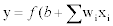(1)

There exist many types of activation functions. If the function is linear, it only transfers the net “n” value to the output unit that is, f (n)=n. This is similar to the linear regression model in econometrics: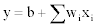(2)

The activation function in most applications however, takes the form of the “log-sigmoid or hyperbolic tangent sigmoid function” which is continuous and nonlinear function and generates the values between 0 and 1, and-1 and +1 respectively. One of the reasons for the popularity of the sigmoid function is that calculating its first derivative, which is needed for weight adjustment in back-propagation, is relatively simple. The sigmoid function is similar to the logit model, where the dependent variable has the logistic functional form. They have the following forms: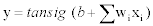(3)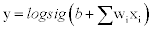(4)

Finally, the output (y) generated by the network is compared with the target or desired output, the error is calculated. The objective is to minimize the error. This is done by applying a “learning” or iteration procedure through which network adjusts the weights (wis) in the direction in which the error is minimized .

Multilayer neural network architecture

The basic single neuron model is very powerful in learning patterns; however, it cannot learn all types of patterns. The multilayer ANN models which have intermediate layer, called the hidden layer, are able to learn all kinds of patterns and thus, are good at prediction. In the multilayer model, the inputs are first processed in the hidden units and the outputs of the hidden units become the inputs of the output units . The output units finally produce the outputs or forecasts. Figure 5 below shows the flow of network processes in the multilayer architecture.

Figure 5: A multilayer ANN with ‘r’ input units, ‘q’ hidden units and one output P P (11) units.

The mathematical processes of the multilayer ANN model are as follows: First, inputs from the input layer enter the network through the hidden layer units. Each hidden layer unit receives the inputs, multiplies them by their correspondent weights and adds them all together with a bias (b). That is, the hidden layer unit (j) calculates: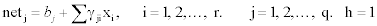(5)

where (xi) is the input of the unit (i) and (γji) is a weight connecting the input unit (i) to the hidden unit (j).The output of the hidden layer unit (j), (Gj), is a transformation of the net, as follows: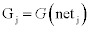(6)

where (G) is an activation function mostly the nonlinear tan-sigmoid function. The output units receive the outputs of the hidden layer units (Gs) as their input. The process in the output layer units is exactly the same as that of the hidden layer units. That is, the output unit calculates the net, the sum of product of the inputs and weights, and bias as follows: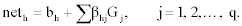(7)

where “neth” is the net value for the output unit (h), (βhj) is the weight connecting the hidden unit (j) to the output unit (h) and (Gj) is the output of the hidden unit (j), which is input for the output unit (h). The output unit then applies a transfer function, (f), to the (neth). The output of the output layer unit (h) is defined as: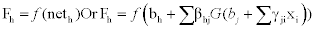(8)

That is, the multilayer ANN mathematical model has the function of function form.

Finally, the network compares the outputs or forecasts (Fs) and the target outputs (Ts), and calculates the error (i.e., Root Mean Squared Forecast Error or RMSFE). The objective is to minimize the error; so, the computed errors are returned to the network in order to adjust the connection weights (γs and βs), hence back-propagation. The weight adjustment process, which is called learning, is done by a specific learning rule. The commonly used learning rule is the “generalized delta rule”. In the delta rule, the weight is updated for each unit in the output layer as follows: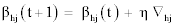(9)

where βhj(t) is the weight connecting unit (j) of the hidden layer to unit (h) of the output layer at time (t), (η) is the learning rate (typically less than 1), and (∇hj ) is the gradient vector associated with the weight (βhj). The gradient vector is the set of derivatives for all weights with respect to the output error . The network calculates the gradient vector on a layer-by-layer basis using the chain rule for partial derivatives. The Levenberg-Marquardt (LM) learning method which is an approximation of Gauss-Newton’s optimization rule is now an improvement of the gradient descent method. It is given as: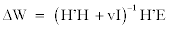(10)

Where (H) is the Jacobian matrix of derivatives of each error to each weight, (v) is the scalar, and (E) is an error vector. The LM update rule approximates gradient descent if (v) is very large, and is equivalent to the Gauss-Newton’s method if (v) is small. In the LM method, (v) changes as the network trains. Since the Gauss-Newton method is faster and more accurate around the minimum error, the network shifts the learning rule from the gradient descent to Gauss-Newton by decreasing v when the error declines. The network iterates the process until the error fails to decrease further, then it stops .

#### Model and Data Specifications

Data type and source

Forecasting models can be constructed with as many predictor variables as possible. From the reviewed literature, money supply growth rate and the exchange rate depreciation are found to be major determinants of the inflation. As such, to estimate and forecast inflation based on the ANN and econometric models, data on the following variables were obtained from the Bank of Ghana: inflation rate, broad money supply (M2+) growth rate and exchange rate depreciation. The data are monthly year-on-year series and cover the period 1991:01 to 2011:12: The data between 1991:01 and 2010:12 are used to estimate the models and that between 2011:01 and 2011:12 are used for the prediction. Figure 6 shows graphically the data used.

Model specification

The main objective of the study is to forecast inflation using the ANN method. However, because the performance of the ANN is compared with econometric models, the ANNs are specified on the basis of the AR and VAR specifications for a fair contest. And so, the econometric models are presented first as follows.

Time-series econometric model: The specified AR and VAR are as follows:

AR (12):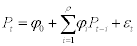(11)

VAR (14):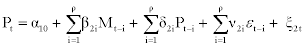(12)

WhereMt−i ,ε tt−i , and Pt−i are past values of: money supply growth, exchange rate and inflation rate respectively and α, β, δ,ϕi and ν are parameters to be estimated.

ANN models: The NAR and NARX models are specified based the AR (12) and VAR (14) models, respectively, in terms of the lag length and variables used. Each ANN model is constructed with twenty (20) hidden layer units and one (1) output layer unit. The ANN transfer functions are also the tan-sigmoid function in the hidden units and the linear function in the output unit. The models are specified as:

NAR (12):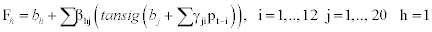(13)

NARX (14):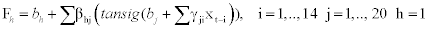(14)

Where xt−i are past values of the input and output variables, γs and βs are hidden and output layer weights respectively and the (bs) are the biases.

ANN model implementation: Designing and implementing neural network model to solve time series problems looks more like an art. However, using the MatLab (2011) neural network toolbox, standard steps are to be followed. The work flow for any of time series problem has the following primary steps.

1. Specify data

2. Create the network

3. Configure the network

4. Initialize the weights and biases

5. Train the network

6. Validate the network

7. Use the network

Data specification: This step identifies the variables that are known to be significant in predicting the target variable. In this case, omitting important variables can affect the network’s performance. For simplicity and reasonable comparison, the ANN models used the same set of variables as in their econometric counterpart .

Creating the network: There are several neural networks for prediction; however, dynamic neural networks are good at time series prediction. The ANN models adopted in this paper are both dynamic neural networks created based on the specification of the time series econometric models presented above. That is, the nonlinear autoregressive (NAR) model and the nonlinear autoregressive with exogenous input (NARX) model were created based on the AR (12) and VAR (14) models, respectively .

Configuring the network: The nonlinearity of the ANN is due to the existence of the hidden layer units. Each network is configured with twenty (20) hidden neurons and one output neuron. The lags are however chosen based on their respective econometric models. The network is also set to randomly divide the input and target output data into three sets as follows: 70% are used for training the network, 15% to measure network generalization, and to halt training when generalization stops improving and the last 15% to provide an independent measure of network performance during and after training [31,32].

Initialising the weights and biases: The weight and biases of the network are randomly initialised. During training these weights and biases are adjusted in order to improve the network performance. A total of 281 weights (parameters) are estimated for the NAR network model and 881 for the NARX model. Hence, the ANNs are largely nonparametric models given the number of parameter estimates and biases [33,34].

Training the network: There are several methods or functions for training ANNs. The Levenberg-Marquardt training algorithm, a standard procedure from the literature, is used in this paper. The network is created and trained in open loop form for efficiency; but, the loop is closed for the prediction [35,36].

Validating the network: The LM training algorithm is run on the training set until the RMSE starts to increase on the validation set. The validated network can then be used for the prediction. Otherwise, the network is retrained with larger data or reconfigured to improve the results [37-39].

Using the network: After training, the network is converted to close loop form where it is used for multi-period forecasting. Finally, the training function produce forecast results on the basis of RMSE minimisation criteria.

Forecast evaluation

To evaluate the forecasting results, the Root Mean of Squared Forecast Error (RMSFE) is calculated as follows: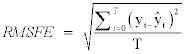(15)

where yt and ŷt are the actual and the forecast values of the dependent variable, and T is the forecast sample size.

#### Empirical Results

The variables for the estimation and forecasting of the models specified in the previous section are first check for stationarity. The ADF test showed that all the series have unit root, implying that they are not stationary; however, their first differences are stationary. The first difference results are shown in Figure 7.

The minimum ACF figure for the first difference of the inflation series occurs at lag twelve (12) and thus the AR (12) model is chosen for the estimation. The AIC criterion also showed that, VAR (14) is the most suitable multivariate model for the series. The estimated AR (12) model had an adjusted R2 of 45% and that of the VAR (14) was 42%. The forecast of the econometric models for the period of 2011 based on the estimated models are shown in Table 1.

Year / Month Actual Inflation AR (12) Forecasts VAR (14) Forecast NAR (12) Forecasts NARX (14) Forecast
2011:01 9.08 8.59 9.13 8.58 8.57
2011:02 9.16 8.78 8.17 9.08 9.08
2011:03 9.13 9.32 10.69 9.16 9.17
2011:04 9.02 9.62 10.38 9.14 9.14
2011:05 8.9 9.37 10.54 9.03 9.04
2011:06 8.59 9.33 10.64 8.91 8.88
2011:07 8.39 8.45 9.06 8.59 8.58
2011:08 8.41 8.38 10.12 8.39 8.38
2011:09 8.4 8.32 9.73 8.42 8.41
2011:10 8.56 8.30 9.80 8.40 8.39
2011:11 8.55 8.73 9.74 8.57 8.55
2011:12 8.58 8.75 8.67 8.56 8.55
MSFE 0.1400 1.6896 0.0377 0.0376
RMSFE 0.3742 1.2999 0.1943 0.1938

Table 1: The summary forecast results of both econometric and ANN models.

The estimated NAR (12) model had an overall R2 of 71% and the NARX had 62%. The two ANN models forecast results for the twelve months of 2011 based on the estimated model are presented in Figure 8. It is clear that the ANN forecast results are very close to the actual results for the same period.

Comparison of the ANN and the econometric models forecast results

The main objective of this paper is to forecast inflation using the ANN method and also to compare the forecast performance of the ANNs with the econometric models on the basis of the RMSFE criterion. The table below shows the summary forecast results of the competing models.

In general, on the basis of the RMSFE criterion, the ANN models have the lowest forecast errors in the one-period-ahead dynamic forecasts between the econometric and ANN models for the forecast period (2011: 01 to 2011:12). Precisely, in the univariate form, the NAR model has lower forecast error compared with its econometric counterpart the AR with the same specification (i.e., with lag length of 12 and the series in their first-differences). Similarly, the NARX model records the lower RMSFE in comparison with the VAR counterpart of the same specification. It is also remarkable to note that, the ANN model in the multivariate form (NARX) slightly outperforms the univariate model (NAR) in the contest. As this may be due to the inclusion of the exogenous inputs to augment the performance of the ANN model, the same cannot be said of the econometric models. Between the econometric time series models, the AR performs significantly better than the multivariate model VAR, implying that the inclusion of the additional independent variables do not improve the forecast performance of the model. In all, the NARX model has the lowest RMSFE and thus is more accurate.

#### Conclusion

This paper used both a univariate and multivariate artificial neural network model to forecast monthly y-o-y inflation in Ghana. The forecast was made for the period 2011 using monthly series from 1991:01 to 2010:12. The main purpose of the work was to generate forecasts that follow closely with actual data using the ANN methodology. The Nonlinear Autoregressive Network (NAR) model and Nonlinear Autoregressive with Exogenous Input Network (NARX) model were each trained with 20 hidden layer units, 1 output unit and LM backpropagation procedure. The forecast results remarkably indicate that both ANNs predict accurately with the NARX producing closer results than the NAR. Finally, the comparison of the out-of-sample forecast performance of the ANNs with their econometric counterparts showed that the RMSFE of the both ANNs are lower than those of AR (12) and VAR (14) models. And so, judging by the RMSFE criterion, forecast based on ANN are more accurate.

#### References

Select your language of interest to view the total content in your interested language

### Article Usage

• Total views: 13722
• [From(publication date):
October-2015 - Aug 19, 2019]
• Breakdown by view type
• HTML page views : 9632
• PDF downloads : 4090

## Post your commentCan't read the image? click here to refresh
###### Peer Reviewed Journals

Make the best use of Scientific Research and information from our 700 + peer reviewed, Open Access Journals

International Conferences 2019-20

Meet Inspiring Speakers and Experts at our 3000+ Global Annual Meetings

Top